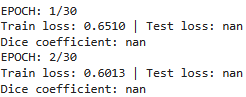# Test loss and dice coefficient giving nan result

i build unet model to segment diabetic retinopathy lesion, but when i did the training with 30 epochs, it give nan value in test loss and dice coefficient

``````startTime = time.time()
batch_size= 2

train_dataset = ProcessDataset(train_x, train_y)
test_dataset = ProcessDataset(test_x, test_y)

num_workers=os.cpu_count(), pin_memory=True)
num_workers=os.cpu_count(), pin_memory=True)

device = torch.device("cuda" if torch.cuda.is_available() else "cpu")
model = Build_Unet()
model.to(device)

trainSteps = len(train_dataset) // batch_size
testSteps = len(test_dataset) // batch_size

H = {"train_loss": [], "test_loss": [], "accuracy": [], "dice": []}

criterion = nn.BCEWithLogitsLoss()
criterion.to(device)

num_epochs = 30

for epoch in range(num_epochs):

model.train()

totalTrainLoss = 0
totalTestLoss = 0

for i, (data, target) in enumerate(train_loader):

data, target = data.to(device), target.to(device)
target = target.unsqueeze(1)

output = model(data)
loss = criterion(output, target)

loss.backward()
optimizer.step()

totalTrainLoss += loss

model.eval()

total_dice = 0

data, target = data.to(device), target.to(device)
target = target.unsqueeze(1)

output = model(data)

loss = criterion(output, target)
totalTestLoss += loss

pred = torch.round(output)

dice = compute_meandice(pred, target, include_background=False)
total_dice += dice

avgTrainLoss = totalTrainLoss / trainSteps
avgTestLoss = totalTestLoss / testSteps

H["train_loss"].append(avgTrainLoss.cpu().detach().numpy())
H["test_loss"].append(avgTestLoss.cpu().detach().numpy())
H["dice"].append(avg_dice)

print("EPOCH: {}/{}".format(epoch + 1, num_epochs))
print("Train loss: {:.4f} | Test loss: {:.4f}".format(
avgTrainLoss, avgTestLoss))
print("Dice coefficient: {:.4f}".format(avg_dice.mean().item()))

endTime = time.time()
print("[INFO] total time taken to train the model: {:.2f}s".format(
endTime - startTime))
``````

here i want to print training loss, test loss, and dice coefficient to see my model performance, but i got stuck in test loss and dice coeficient giving nan value like thissince i’m newbie in pytorch, anyone know how to fix it? or is there something strange in my code? thankyou in adanvance!

Hi Anastasia!

First some context: `nan` is a “special” floating-point number. It means
“not a number.” It appears as the result of certain ill-defined mathematical
operations such as zero divided by zero or infinity minus infinity.

It also has the property that any operation on a `nan` will result in another
`nan`.

So, if an element of one of the weights in your model is `nan`, then the
output of your model will (in most cases) be `nan`.

Now some suggestions on how to track down the source of your `nan`:

That your `train loss` is not `nan` suggests that your model doesn’t have
any `nan`s in it.

So first check that your test data (that you input to your model) doesn’t
have any `nan`s in it. If not, then start passing samples from `test_dataset`
through your model and check whether the `output` has any `nan`s. Note
that any single `nan` in the `output` from any sample from `test_dataset`
will result in `test_loss` becoming `nan`.

Beware that if `len (test_dataset)` is less than `batch_size` then
`testSteps = len(test_dataset) // batch_size` will round down to
zero, and `avgTestLoss = totalTestLoss / testSteps` will be `0.0 / 0`
which will be `nan`.

Try these steps to see if you can locate where the `nan`s are creeping in
and feel free to post any follow-up questions.

Good luck!

K. Frank

1 Like

hi, thankyou for your time. you suggest to check my test data, how can i check that? my test dataset is contains of 27 images, so i assume that testSteps won’t be round down to zero. could you provide me some solution? thanks!

Hi Anastasia!

You could do something like:

``````            if  data.isnan().any():
print ('found a nan in data.')
output = model(data)
if  output.isnan().any():
print ('found a nan in output.')
``````

and do the same for `target` and `loss`, if necessary.

Best.

K. Frank

hi, thanks for your advice! now my test loss already had a value, but my dice coefficient still nan. i try to do your advice but it didn’t print anything so i assume that my data and target are definitely okay.

97%|█████████▋| 29/30 [02:02<00:04, 4.31s/it]

EPOCH: 29/30 Train loss: 0.0289 | Test loss: 0.0508 Dice coefficient: nan

100%|██████████| 30/30 [02:06<00:00, 4.21s/it]

EPOCH: 30/30 Train loss: 0.0299 | Test loss: 0.0442 Dice coefficient: nan

Hi Anastasia!

The Dice coefficient is a normalized count of the number of true positives
your model predicts. If neither your ground truth (your `target`) nor your
(rounded) predictions (your `pred`) contain any positives for the sample
(or batch of samples) in question, then the formula for the Dice coefficient
will give `0 / 0` which will become `nan`.

If your function `compute_meandice()` doesn’t protect against such a
possibility, then it could return `nan` and pollute your running Dice score,
`total_dice`.

If this doesn’t happen very often, you could just use `0.0` for the value
of `dice` when `nan` occurs. If it happens with some frequency, you might
want to leave such samples out of your `avg_dice` computation:

``````avg_dice = total_non_nan_dice / count_non_nan_dice
``````

Best.

K. Frank

ah thanks! after i rechecked, it is because centercropping that crop the mask, so the mask is just black background